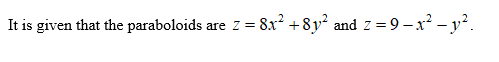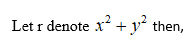# Use polar coordinates to find the volume of the given solid.Bounded by the paraboloids z = 8x2 + 8y2 and z = 9 − x2 − y2

Question
258 views

Use polar coordinates to find the volume of the given solid.

Bounded by the paraboloids z = 8x2 + 8y2 and z = 9 − x2 − y2
check_circle

Step 1Step 2

Equate the paraboloids as,

Step 3...

### Want to see the full answer?

See Solution

#### Want to see this answer and more?

Solutions are written by subject experts who are available 24/7. Questions are typically answered within 1 hour.*

See Solution
*Response times may vary by subject and question.
Tagged in

### Math